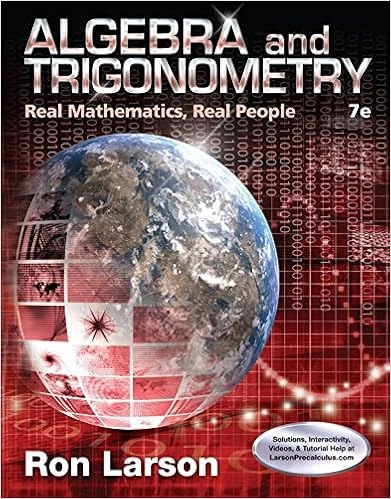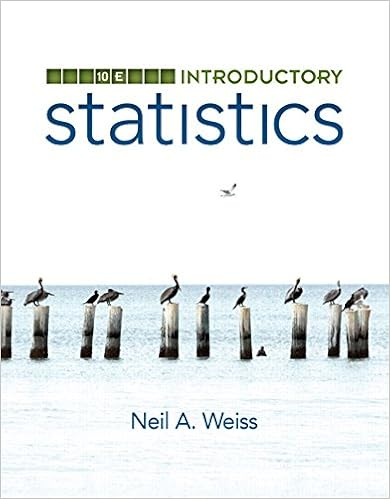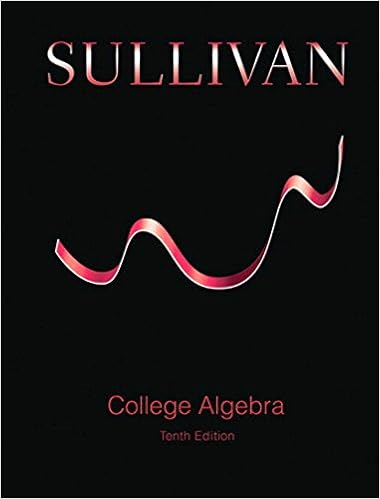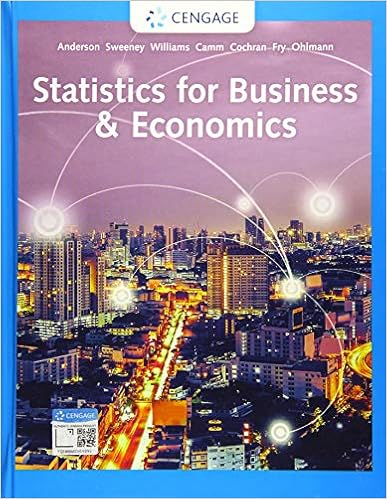# Topic 1 Algebraic Expressions - Olympic College Topic 1...

• Notes
• 22

This preview shows page 1 - 4 out of 22 pages.

Olympic College Topic 1Algebraic ExpressionsPage | 1Algebraic Expressions1. Introduction:Definition:In algebra, we use letters such as x, y and z to represent unknown numbers.For example 5x + 4y an algebraic expression. In this expression we call x the variable and 5is called the coefficient of x. We can treat mathematical expressions in the same way asnumbers provided we obey certain rules of algebra. We can also translate information that isgiven in English into an equivalent mathematical form using algebraic expressions?If a statement is given in English that uses the four basic arithmetic operations of addition,subtraction, multiplication and division we can translate this information into an equivalentalgebraic expression.For example.p multiplied by 3 can be written as the expression 3p11 added to x can be written as the expression 11 + x8 subtracted from r can be written as the expression r8m multiplied by 4 can be written as the expression 4m4 multiplied by m can be written as the expression 4mx divided by 4 can be written as the expression4 divided by x can be written as the expressionWhen you combine more than one variable you can also express the information as algebraicexpressionsFor example.x added to y can be written as the expression x + yx subtracted from y can be written as the expression yxa multiplied by b can be written as the expression ab4 multiplied by m squared can be written as the expression 4m2x divided by y can be written as
##### We have textbook solutions for you!The document you are viewing contains questions related to this textbook.
Chapter 9 / Exercise 6
Algebra and Trigonometry: Real Mathematics, Real People
LarsonExpert VerifiedBrowse all Textbook Solutions
Olympic College Topic 1Algebraic ExpressionsExercise 1A:Express each of the following statements as and algebraic expression.1.p multiplied by 102.5 added to x3.3 subtracted from t4.b multiplied by 25.a multiplied by c6.p divided by q7.r squared8.z cubed9.a added to d10.12 multiplied by r11.n squared added to b12.5 divided by h13.8 multiplied by x14.4 multiplied by m squared15.w divided by e16.7 subtracted from q17.r squared take away 318.y to the power 919.d to the power 820.30 multiplied by u plus 4Exercise 1B:1.Write an algebraic expression for the phrase 2 times the quantityqminus 3.A.2q3B. 2(q3)C.32.Write an algebraic expression for the phrase 4 subtract x add yA.x4 + yB. 4xyC.4x + yD.3.Write an algebraic expression for the phrase x subtracted from y and the result isdivided by 52qD.4 + x + yD.
Page | 2
4.Write an algebraic expression for the product of w and d plus 3 times g
5.Write an algebraic expression for the phrase square the quantity x and then double it.)
Olympic College Topic 1Algebraic ExpressionsPage | 3

Upload your study docs or become a

Course Hero member to access this document

Upload your study docs or become a

Course Hero member to access this document

End of preview. Want to read all 22 pages?

Upload your study docs or become a

Course Hero member to access this document

Term
Fall
Professor
DRobertson
Tags
Algebraic Expressions, Velocity, Olympic College Topic
##### We have textbook solutions for you!
The document you are viewing contains questions related to this textbook.The document you are viewing contains questions related to this textbook.
Chapter 9 / Exercise 6
Algebra and Trigonometry: Real Mathematics, Real People
LarsonExpert Verified
•••